# Abductive reasoning

Abduction, or inference to the best explanation, is a method of reasoning in which one chooses the hypothesis that would, if true, best explain the relevant evidence. Abductive reasoning starts from a set of accepted facts and infers most likely, or best, explanations. The term "abduction" also sometimes only refers to the generation of hypotheses that explain observations or conclusions, but the former definition is more common both in philosophy and computing.

Aristotle discussed abductive reasoning (apagoge, Greek) in his Prior Analytics. Charles Peirce formulated abduction as a method of scientific research and introduced it into modern logic. The concept of abduction is applied beyond logic to the social sciences and the development of artificial intelligence.

## Logical reasoning: Deduction, induction, and abduction

There are three kinds of logical reasoning in logic: Deduction, induction, and abduction. Given a precondition, a conclusion, and a rule that the precondition implies the conclusion, they can be explained in the following way:

• Deduction means determining the conclusion. It is using the rule and its precondition to make a conclusion. Example: "When it rains, the grass gets wet. It rains. Thus, the grass is wet." Mathematicians are commonly associated with this style of reasoning.
It allows deriving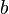$b$ as a consequence of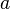$a$. In other words, deduction is the process of deriving the consequences of what is assumed. Given the truth of the assumptions, a valid deduction guarantees the truth of the conclusion. A deductive statement is based on accepted truths. For example, all bachelors are unmarried men. It is true by definition and is independent of sense experience.
• Induction means determining the rule. It is learning the rule after numerous examples of the conclusion following the precondition. Example: "The grass has been wet every time it has rained. Thus, when it rains, the grass gets wet." Scientists are commonly associated with this style of reasoning.
It allows inferring some$a$ from multiple instantiations of$b$ when$a$ entails$b$. Induction is the process of inferring probable antecedents as a result of observing multiple consequents. An inductive statement requires perception for it to be true. For example, the statement, "it is snowing outside" is invalid until one looks or goes outside to see whether it is true or not. Induction requires sense experience.
• Abduction means determining the precondition. It is using the conclusion and the rule to assume that the precondition could explain the conclusion. Example: "When it rains, the grass gets wet. The grass is wet, it must have rained." Diagnosticians and detectives are commonly associated with this style of reasoning.
It allows inferring$a$ as an explanation of$b$. Because of this, abduction allows the precondition$a$ of “$a$ entails$b$” to be inferred from the consequence$b$. Deduction and abduction thus differ in the direction in which a rule like “$a$ entails$b$” is used for inference. As such abduction is formally equivalent to the logical fallacy affirming the consequent or Post hoc ergo propter hoc, because there are multiple possible explanations for$b$.

Unlike deduction and induction, abduction can produce results that are incorrect within its formal system. However, it can still be useful as a heuristic, especially when something is known about the likelihood of different causes for$b$.

## Logic-based abduction

In logic, an explanation is expressed by$T$, which represents a domain and a set of observations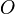$O$. Abduction is the process of deriving a set of explanations of$O$ according to$T$ and picking out one of those explanations. For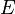$E$ to be an explanation of$O$ according to$T$, it should satisfy two conditions:

•$O$ follows from$E$ and$T$;
•$E$ is consistent with$T$.

In formal logic,$O$ and$E$ are assumed to be sets of literals. The two conditions for$E$ being an explanation of$O$ according to theory$T$ are formalized as: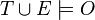$T \cup E \models O$;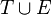$T \cup E$ is consistent.

Among the possible explanations$E$ satisfying these two conditions, some other condition of minimality is usually imposed to avoid irrelevant facts (not contributing to the entailment of$O$) being included in the explanations. Abduction is then the process that picks out some member of$E$. Criteria for picking out a member representing "the best" explanation include the simplicity, the prior probability, or the explanatory power of the explanation.

A proof theoretical abduction method for first order classical logic based on sequent calculus and dual calculus, based on semantic tableaux (analytic tableaux) has been proposed. The methods are sound and complete, and they work for full first order logic without requiring any preliminary reduction of formulae into normal forms. These methods have also been extended to modal logic.

Abductive logic programming is a computational framework that extends normal logic programming with abduction. It separates the theory$T$ into two components, one of which is a normal logic program, used to generate$E$ by means of backward reasoning, the other of which is a set of integrity constraints, used to filter the set of candidate explanations.

## Set-cover abduction

A different formalization of abduction is based on inverting the function that calculates the visible effects of the hypotheses. Formally, one is given a set of hypotheses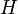$H$ and a set of manifestations$M$; they are related by the domain knowledge, represented by a function$e$ that takes as an argument a set of hypotheses and gives as a result the corresponding set of manifestations. In other words, for every subset of the hypotheses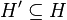$H' \subseteq H$, their effects are known to be$e(H')$.

Abduction is performed by finding a set$H' \subseteq H$ such that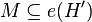$M \subseteq e(H')$. In other words, abduction is performed by finding a set of hypotheses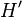$H'$ such that their effects$e(H')$ include all observations$M$.

A common assumption is that the effects of the hypotheses are independent, that is, for every$H' \subseteq H$, it holds that$e(H') = \bigcup_{h \in H'} e(\{h\})$. If this condition is met, abduction can be seen as a form of set covering.

## History of the concept

Historically, Aristotle's use of the term "epagoge" has referred to a syllogism in which the major premise is known to be true, but the minor premise is only probable.

The philosopher Charles Peirce introduced abduction into modern logic. In his works before 1900, he mostly uses the term to mean the use of a known rule to explain an observation; for example, “if it rains the grass is wet,” is a known rule used to explain that the grass is wet. In other words, it would be more technically correct to say, "If the grass is wet, the most probable explanation is that it recently rained."

He later used the term to mean creating new rules to explain new observations, emphasizing that abduction is the only logical process that actually creates anything new. Namely, he described the process of science as a combination of abduction, deduction, and induction, stressing that new knowledge is only created by abduction.

This is contrary to the common use of abduction in the social sciences and in artificial intelligence, where the old meaning is used. Contrary to this use, Peirce stated that the actual process of generating a new rule is not “hampered” by logic rules. Rather, he pointed out that humans have an innate ability to infer correctly; possessing this ability is explained by the evolutionary advantage it gives. Peirce's second use of "abduction" is most similar to induction.

Norwood Russell Hanson, a philosopher of science, wanted to grasp a logic that explained how scientific discoveries take place. He used Peirce's notion of abduction for this.

Further development of the concept can be found in Peter Lipton's Inference to the Best Explanation.

## Applications

Applications in artificial intelligence include fault diagnosis, belief revision, and automated planning. The most direct application of abduction is that of automatically detecting faults in systems: Given a theory relating faults with their effects and a set of observed effects, abduction can be used to derive sets of faults that are likely to be the cause of the problem.

Abduction can also be used to model automated planning. Given a logical theory relating action occurrences with their effects (for example, a formula of the event calculus), the problem of finding a plan for reaching a state can be modeled as the problem of abducting a set of literals implying that the final state is the goal state.

Belief revision, the process of adapting beliefs in view of new information, is another field in which abduction has been applied. The main problem of belief revision is that the new information may be inconsistent with the corpus of beliefs, while the result of the incorporation cannot be inconsistent. This process can be done by the use of abduction: Once an explanation for the observation has been found, integrating it does not generate inconsistency. This use of abduction is not straightforward, as adding propositional formulae to other propositional formulae can only make inconsistencies worse. Instead, abduction is done at the level of the ordering of preference of the possible worlds.

In the philosophy of science, abduction has been the key inference method to support scientific realism, and much of the debate about scientific realism is focused on whether abduction is an acceptable method of inference.

In historical linguistics, abduction during language acquisition is often taken to be an essential part of processes of language change such as reanalysis and analogy.

## Abductive validation

Abductive validation is the process of validating a given hypothesis through abductive reasoning. Under this principle, an explanation is valid if it is the best possible explanation of a set of known data. The best possible explanation is often defined in terms of simplicity and elegance (such as Occam's razor). Abductive validation is common practice in hypothesis formation in science.

After obtaining results from an inference procedure, we may be left with multiple assumptions, some of which may be contradictory. Abductive validation is a method for identifying the assumptions that will lead to a goal.